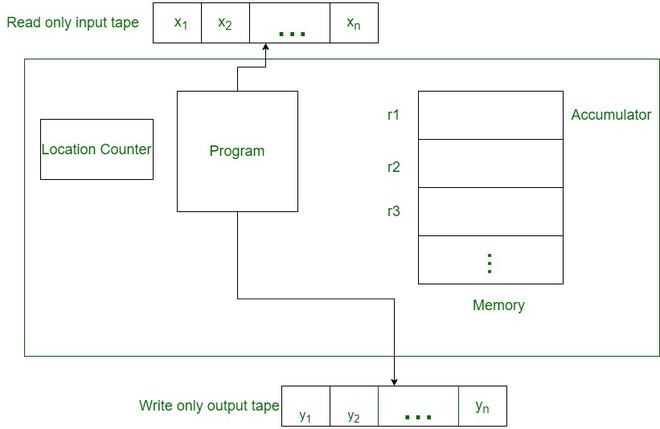GFG App
Open AppBrowser
Continue

The algorithm required to solve a problem on a sequential computer is called a sequential algorithm. Algorithms written to solve a problem on a parallel computer are called parallel algorithms. The sequential algorithm is written in the form of steps, which are done sequentially by PE, i.e. sequential algorithm uses sequential steps to solve a problem. In a parallel algorithm, we have to see where the communication is taking place, where we have to move the output of particular steps.

Algorithms written for a particular architecture cannot be applied to another architecture, in other words, to solve the same problem on different parallel computers, different algorithms must be written based on parallel computer architecture.

Writing parallel algorithms is a complex task, to deal with this problem various parallel models called abstract machines were designed hypothetically.

Abstract machines are just imaginary notions, they don’t exist in reality. Here we can make inferences to solve a problem.

There are two types of Abstract Machine

#### Random Access Machine (RAM)

Random Access Machine or RAM model is a CPU. It is a potentially unbound bank of memory cells, each of which can contain an arbitrary number or character. Memory cells are numbered and it takes time to access any cell in memory or say all operations (read/write from memory, standard arithmetic, and Boolean operations) take a unit of time. RAM is a standard theoretical model of computation (infinite memory and equal access cost). The Random Access Machine model is critical to the success of the computer industry.Random Access Machine

• The Random Access Machine (RAM) model is a one address computer.
• RAM is a sequential machine. RAM consists of memory, read-only input tape, output tape only, and writes a program.
• The program is not stored in memory and cannot be modified.
• The input tape consists of a series of integers. Each time an input value is read, the input head moves forward one square.
• Similarly, the output head moves forward after each write. Memory consists of an infinite sequence of registers, designated r0, r1, r2,….
• Each register can contain an integer. Register r0 is the accumulator, where the calculation is done.
• RAM must have the same instructions as LOAD, STORE, READ, WRITE, ADD, SUBTRACT, MULTIPLY, DIVIDE, TEST, JUMP, and HALT.
• The worst-case time complexity function of a RAM program is f(n), which is the maximum time taken by the program to execute on all inputs of size n.
• We assume that each of these steps takes a constant, that is, O(1) time.

In RAM, Here each step of an algorithm consists of the following steps:

• Read: (Up to) N processors simultaneously (in parallel) read from N memory locations (as in memory) and store the values in their local registers.
• Compute: (Up to) N processors perform basic arithmetic or logical operations on values in their registers.
• Write: (Up to) N processors simultaneously write from their registers in (up to) N memory locations.

Each step, READ, COMPUTE, WRITE, is assumed to take O(1) time in the case of RAM. It should be noted that not all processors need to perform a given step of the algorithm. When a subset of processors takes a step, other processors remain idle during that time. Algorithms for PRAM have to specify which subset of processors should be active during the execution of a phase.

My Personal Notes arrow_drop_up